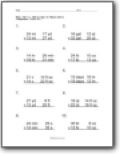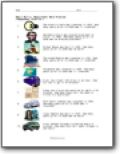# Operations With Units of Measure Worksheets

#### How to Add and Subtract Units of Measure

We are already familiar with the concept of addition and subtraction of normal numbers. However, as we move to measure units, the method becomes more customized. It would help if you always remembered that only the same kinds of units could be added or subtracted. In other words, you cannot add two chairs and three tables together. You can only add 2 chairs and 3 chairs to make them 5 chairs. In many cases, you will need to convert units of measure to the same system and exact denomination within that system. Once you have completed that it is only a matter of processing the operations. You just need to pay attention to the subunits of measurement system to make sure that they units do not denature some where along the way. The best way to approach this is act like the difference between denominations are place values and then carry on.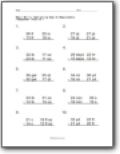###### Subtracting English Measurements

Students are given random English measures and asked to state the difference between them.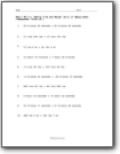###### Adding Time and Weight Units of Measurement

Students find the sum of two measures that are either units of time or mass/weight.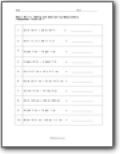We use a wide variety of measures to practice finding differences and sums.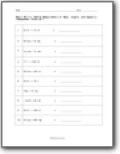###### Adding Measurements of Mass, Length, and Capacity

Find the sum of all these measures. Make certain that you convert properly before processing the operation.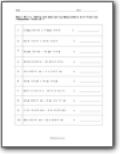###### Adding and Subtracting Measurements with Fractions

You will process all these operations that include the use of fractional units.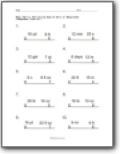###### Multiplying English Units of Measurement

These problems are all set up as if you working in construction and completed a project that require a set value of supplies. You need to calculate how many more you would need based on the multiplier that is presented to you.